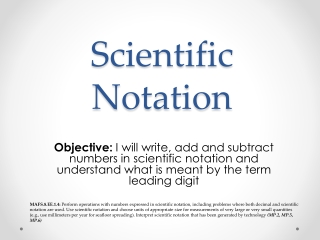DownloadDownload PresentationScientific Notation

# Scientific Notation

Download Presentation## Scientific Notation

- - - - - - - - - - - - - - - - - - - - - - - - - - - E N D - - - - - - - - - - - - - - - - - - - - - - - - - - -
##### Presentation Transcript

1. Scientific Notation Objective: I will write, add and subtract numbers in scientific notation and understand what is meant by the term leading digit MAFS.8.EE.1.4: Perform operations with numbers expressed in scientific notation, including problems where both decimal and scientific notation are used. Use scientific notation and choose units of appropriate size for measurements of very large or very small quantities (e.g., use millimeters per year for seafloor spreading). Interpret scientific notation that has been generated by technology (MP.2, MP.5, MP.6)

2. Do Now Problem: Most English-speaking countries use the short-scale naming system, in which a trillion is expressed as . Some other countries use the long-scale naming system, in which a trillion is expressed as . Express each number as a single-digit integer times a power of ten. How many times greater is the long-scale naming system than the short-scale?

3. Scientific Notation Consider the estimated number of stars in the universe: . This is a 23-digit whole number with the leading digit (the leftmost digit) 6 followed by 22 zeroes A positive, finite decimal is said to be written in scientific notation if it is expressed as a product , where is a finite decimal so that , and is an integer

4. Scientific Notation The finite decimal 234.567 is equal to every one of the following:

5. Scientific Notation Are the following numbers written in scientific notation? If not, state why.

6. Scientific Notation • Let’s say we need to determine the difference in the populations of Texas and North Dakota. In 2012, Texas had a population of about million people and North Dakota had a population of about : • We begin by writing each number in scientific notation: • Texas: • North Dakota: • To find the difference, we subtract:

7. Scientific Notation By the First Law of Exponents By the Distributive Property

8. Scientific Notation • Find the combined mass of two hydrogen atoms and one oxygen atom, which is normally written as H2O or otherwise known as “water.” • One hydrogen atom is approximately 0.000 000 000 000 000 000 000 000 001 7 kilograms. • One oxygen atom is approximately 0.000 000 000 000 000 000 000 000 027 kilograms.

9. Scientific Notation • First, we should write each atom in scientific notation: • Hydrogen: • Oxygen: • 2 Hydrogen atoms = • 2 Hydrogen atoms + 1 Oxygen atom = Then we change them both into 10-26: By the First Law of Exponents By the Distributive Property